Polynomials Approximation Method for Solving Parabolic Partial Differential Equations | OMICS International
Journal of Generalized Lie Theory and Applications

# Polynomials Approximation Method for Solving Parabolic Partial Differential Equations

Sunday Babuba*

Federal University Dutse, Ibrahim Aliyu Bye-Pass, P.M.B. 7156, Dutse, Jigawa State – Nigeria

*Corresponding Author:
Sunday Babuba
Federal University Dutse
Ibrahim Aliyu Bye-Pass
P.M.B. 7156, Dutse
Jigawa State – Nigeria
Tel: +2348039282881
E-mail: [email protected]

Received Date: June 12, 2017; Accepted Date: July 26, 2017; Published Date: July 30, 2017

Citation: Babuba S (2017) Polynomials Approximation Method for Solving Parabolic Partial Differential Equations. J Generalized Lie Theory Appl 11: 277. doi: 10.4172/1736-4337.1000277

Copyright: © 2017 Babuba S. This is an open-access article distributed under the terms of the Creative Commons Attribution License, which permits unrestricted use, distribution, and reproduction in any medium, provided the original author and source are credited.

Visit for more related articles at Journal of Generalized Lie Theory and Applications

#### Abstract

A new numerical method based on the approximation of polynomials is here proposed for solving the one dimensional parabolic partial differential equation arising from unsteady state flow of heat subject to initial and boundary conditions. The method results from discretization of the parabolic partial differential equation which leads to the production of a system of algebraic equations. By solving the system of algebraic equations we obtain the problem approximate solutions.

#### Keywords

Polynomials; Interpolation; Collocation; Parabolic

#### Introduction

The development of continuous numerical techniques for solving parabolic partial differential equations in physics and mathematics subject to initial and boundary conditions is a subject of considerable interest. In this paper, we develop a new numerical method based on interpolation and collocation of the equation arising from approximating the polynomials [1-27]. To do this, we let U(x,t) represent the temperature at any point in a rod, whose distance from one end is x and X is the length of the rod. Heat is flowing from one end to another under the influence of the temperature gradient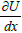. To make a balance of the rate of heat flow in and out of the element, we consider R for thermal conductivity and c the heat capacity which we assume constants, and ρ the density and t represent the time coordinate. In this study we try to find solution to the heat flow equation: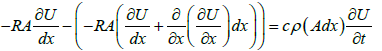(1)

Where A is the cross section of the rod and the initial and boundary conditions are given by:

U (x,t ) =U (x,0) = f (x) and U (0,t) = c1,U ( X ,0) = c2 , where c1, c2 are constants.

#### The Solution Method

To set up the solution method we select an integer N such that N>0. We subdivide the interval 0≤xX into N equal subintervals with meshes given by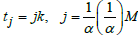where Nh=X. Similarly, we reverse the roles of x and t and we select an integer M such that M>0. We also subdivide the interval 0≤tT into M equal subintervals with meshes given by, where Mk=T, and h,k are the mesh sizes along space and time coordinates. We seek for the approximate solution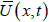to U(x,t) of the form: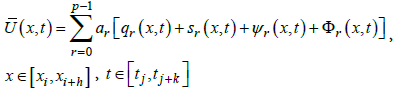(2)

over h>0, k>0 successive subintervals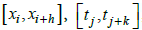, such that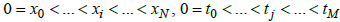. p is the sum of interpolation points along the space and time coordinates respectively. That is p=g+b, where g is the number of interpolation points along the space coordinate, while b is the number of interpolation points along the time coordinate. The bases functions qr , sr ψ r , Φr , r = 0,1,..., p −1 are the Taylor, Legendre, chebyshev and Laguerre polynomials which are known, ar are the constants to be determined. The interpolation values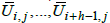are assumed to have been determined from previous steps, while the method seeks to obtain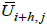[5-26]. Expanding eqn. (2) we obtain: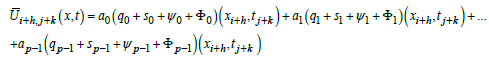(3)

We let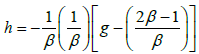, where g is the number of interpolation points along the space coordinate. Then, by Cramer’s rule we obtain from eqn. (3),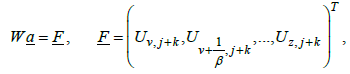(4)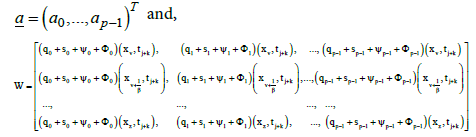Where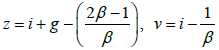and W−1 exist [10-27].

Hence, by eqn. (4), we obtain: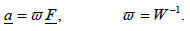(5)

The vector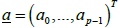is now determined in terms of known parameters in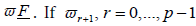is the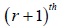row ofϖ, then,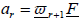(6)

Eqn. (6) determines the values of ar, r=0,1,...,p−1.

We take first and second derivatives of eqn. (2) with respect to x,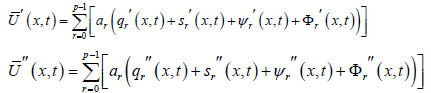(7)

Putting eqn. (6) in eqn. (7) we obtain: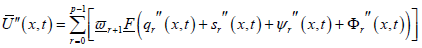(8)

We reverse the roles of x and t in eqn. (3) and we set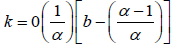, where b is the number of interpolation points along the time coordinate, then by Cramer’s rule we obtain,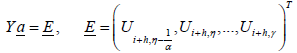(9)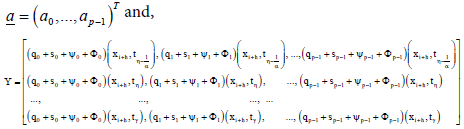where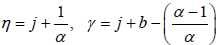and Y−1 exist [1-16].

Hence, from eqn. (9) we obtain: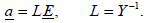(10)

The vector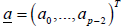is now determined in terms of known parameters in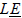.

If Lr+1, r=0,...,p−1 is the (r+1)th row of L then,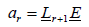(11)

Also, eqn. (11) determines the values of ar, r=0,1,...,p−1.

Taking the first derivatives of eqn. (2) with respect to t we obtain: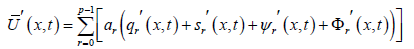(12)

Putting eqn. (11) in eqn. (12) we obtain: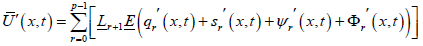(13)

But by eqn. (1), it is obvious that eqn. (13) is equal to eqn. (8), therefore,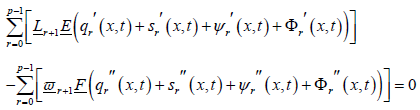(14)

If we collocate eqn. (14) at x=xi and t=tj we obtain a new numerical scheme that solves eqn. (1) explicitly.

#### Numerical Examples

In this section, we will test the numerical accuracy of the new method by using the new scheme to solve four (4) test examples. That is, we compute an approximate solution of eqn. (1) at each time level. To achieve this, we truncate the polynomials after second degree. We now specifically use the resultant scheme to solve examples 4.1, 4.2, 4.3 and 4.4 below.

Example 4.1

Solve for the temperatures in a copper rod 1cm long, with the outer curved surface insulated so that heat flows in only one direction. If the initial temperature (°C) within the rod is given as a function of the distance from one face by the equation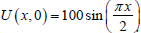. Find the temperatures as a function of x and t if both faces are maintained at (°C). Copper has a thermal conductivity A=0.13cal/sec.cm. °C, its heat capacity c=0.11cal.g. °C and density ρ=7.8g/cm3

To solve example 3.1, therefore, we simplify eqn. (1) and apply eqn. (16) to solve it.

By simplification eqn. (1) becomes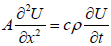. To solve this equation, we take Δx=0.25cm, then we find Δt by the relation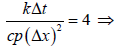, Δt = 28.78sec. we also take β=4, α=128, it implies that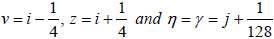. We take two interpolation points along space coordinate and one interpolation point along time coordinate. This implies that g=2, b=1, and p=3. Therefore, for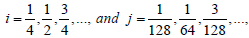implies that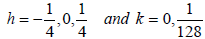, then the calculated temperatures and concentrations of alcohol are as shown below Table 1.

 t x=0 x=0.25 x=0.50 x=0.75 x=1.00 x=1.25 0.00 0.00 38.27 70.71 92.39 100 92.39 28.78 0.0 37.54 69.37 90.63 98.10 90.63 57.57 0.0 36.83 68.05 88.91 96.23 88.91 86.35 0.0 36.13 66.76 87.23 94.40 87.23 115.51 0.0 35.45 65.49 85.59 92.61 85.59 143.91 0.0 34.77 64.24 83.94 90.85 83.94

Table 1: Calculated temperatures.

Example 4.2

A hollow tube 20 cm long is initially filled with air containing 2% of ethyl alcohol vapors. At the bottom of the tube is a pool of alcohol which evaporates into the stagnant gas above. (Heat transfers to the alcohol from the surroundings to maintain a constant temperature of 30°C, at which temperature the vapor pressure is 0.1 atm.) At the upper end of the tube, the alcohol vapors dissipate to the outside air, so the concentration is essentially zero. Considering only the effects of molecular diffusion, determine the concentration of alcohol as a function of time and the distance x measured from the top of the tube. Molecular diffusion follows the law: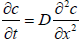, where D is the diffusion coefficient, with units of cm2/sec. (This is the same as for the ratio k / cp , which is often termed thermal diffusivity.) For ethyl alcohol, D=0.102 cm2/sec at 30°C, and the vapor pressure is such that 10 volume percent alcohol in air is present at the surface. Our initial condition is c(x,0)=2.0. The boundary conditions are c(0,t)=0, c(20,t)=10. To solve this resulting equation we subdivide the length of the tube into five intervals, such that Δx=4cm. Using the maximum value permitted for Δt yields: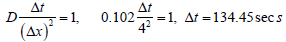. We also take β=4, α==32, it implies that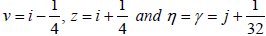.

We take two interpolation points along space coordinate and one interpolation point along time coordinate. This implies that g = 2, b = 1, and p = 3 . For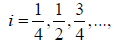and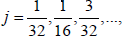this implies that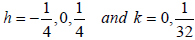, then the calculated concentrations of alcohol in Table 2.

 t x=0 x=4 x=8 x=12 x=16 x=20 0.0 0.0 2 2 2 2 10 134.45 0.0 1.75 2 2 6 10 268.90 0.0 1.56 1.97 3.50 7 10 403.35 0.0 1.42 2.11 3.75 6.94 10 537.80 0.0 1.33 2.23 3.94 6.92 10 672.25 0.0 1.28 1.67 4.10 6.93 10

Table 2: Calculated concentrations of alcohol.

Example 4.3

Use the scheme to approximate the solution to the heat equation (Table 3).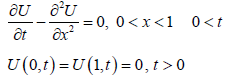U (x,0) = sinπ x, 0 ≤ x ≤1

x Computed solution U(x,t) Exact solution U(x,t) Schmidt Method U(x,t) Errors
New Method Schmidt Method
0 0 0 0 0 0
0.1 0.308008706 0.308002141 0.307963277 6.6 X E-6 2.1 X E-4
0.2 0.585867367 0.585854886 0.58577788 1.2 X E-5 4.0 X E-4
0.3 0.806377253 0.806360073 0.806254085 1.7 X E-5 5.6 X E-4
0.4 0.947953314 0.947932118 0.947808521 2.0 X E-5 6.6 X E-4
0.5 0.996737101 0.996715865 0.996584857 2.1 X E-5 1.2 X E -4
0.6 0.947953314 0.947932118 0.947808521 2.0 X E-5 6.6 X E-4
0.7 0.806377253 0.806360073 0.806254085 1.7 X E-5 5.6 X E-4
0.8 0.585867367 0.585854886 0.58577788 1.2 X E-5 4.0 X E-4
0.9 0.398221058 0.308002141 0.307963277 6.6 X E-6 2.1 X E-4
1.0 0 0 0 0 0

Table 3: Result of action of the scheme on problem.

Example 4.4

Use the scheme to approximate the solution to the heat equation (Table 4).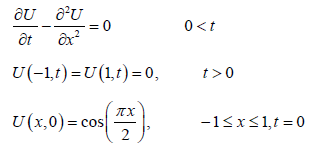x Exact solution U(x,t) Computed solution U(x,t) Schmidt Method Errors
New Method Schmidt Method
0 0 0 0 0 0
0.1 0.308008706 0.308002141 0.307963277 6.6 X E-6 2.1 X E-4
0.2 0.585867367 0.585854886 0.58577788 1.2 X E-5 4.0 X E-4
0.3 0.806377253 0.806360073 0.806254085 1.7 X E-5 5.6 X E-4
0.4 0.947953314 0.947932118 0.947808521 2.0 X E-5 6.6 X E-4
0.5 0.996737101 0.996715865 0.996584857 2.1 X E-5 1.2 X E -4
0.6 0.947953314 0.947932118 0.947808521 2.0 X E-5 6.6 X E-4
0.7 0.806377253 0.806360073 0.806254085 1.7 X E-5 5.6 X E-4
0.8 0.585867367 0.585854886 0.58577788 1.2 X E-5 4.0 X E-4
0.9 0.398221058 0.308002141 0.307963277 6.6 X E-6 2.1 X E-4
1.0 0 0 0 0 0

Table 4: Result of action of the new scheme on problem.

#### Conclusion

In this work, I proposed a method to find the solution of the system of ordinary differential equations arising from discretizing the parabolic partial differential equations with respect to space variables. Discretization of many of the partial differential equations result in this type of the systems and the proposed method can be applied for computation the solutions of the systems.

#### References

Select your language of interest to view the total content in your interested language

### Article Usage

• Total views: 1586
• [From(publication date):
August-2017 - Dec 09, 2019]
• Breakdown by view type
• HTML page views : 1452Can't read the image? click here to refresh